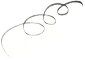# Kalkules Matrix Calculator

by Jardo

## Kalkules is a free, feature-rich scientific calculator designed to be easy to use while providing powerful features.

Operating system: Windows

Publisher: Jardo

Antivirus check: passed

Last revision: Last week

Report a Problem

Kalkules Matrix Calculator

Kalkules Matrix Calculator is a powerful and versatile software solution for performing numerical calculations with matrices. It is designed to be easy to use for mathematicians of all levels, from students to professionals. With Kalkules Matrix Calculator, users can perform a variety of calculations, such as matrix addition, subtraction, multiplication, and division, with multiple matrix types, including real, complex, and quaternionic matrices. It also offers an easy-to-use graphical user interface, which makes it simple to input, change, and view data.

Kalkules Matrix Calculator provides a simple and intuitive interface for performing complex calculations with ease.
Features:

• Matrix Types: Kalkules Matrix Calculator supports real, complex, and quaternionic matrices.

• Graphical User Interface: The intuitive graphical user interface makes it easy to input, change, and view data.

• Matrix Calculations: Kalkules Matrix Calculator offers a variety of calculations, including matrix addition, subtraction, multiplication, and division.

• Symbolic Calculations: Kalkules Matrix Calculator can also handle symbolic calculations, such as finding the inverse of a matrix.

• Eigenvalue and Eigenvector Calculations: Kalkules Matrix Calculator can calculate the eigenvalues and eigenvectors of a matrix.

• Polynomial Calculations: Kalkules Matrix Calculator can also perform polynomial calculations, such as finding the roots of a polynomial.

• Vector Calculations: Kalkules Matrix Calculator can also perform vector calculations, such as calculating the dot product and cross product of two vectors.

• Plotting: Kalkules Matrix Calculator can also plot functions and matrices to help users visualize their data.

• File Support: Kalkules Matrix Calculator supports a variety of file formats, including .csv, .txt, .xlsx, and .mat.

• Customization: Kalkules Matrix Calculator allows users to customize their calculations with a variety of settings, such as setting the precision of the results.

• Documentation: Kalkules Matrix Calculator comes with a comprehensive user guide and online support to help users get the most out of the software.

• Compatibility: Kalkules Matrix Calculator is compatible with Windows, Mac, and Linux operating systems.

Kalkules Matrix Calculator is a powerful and versatile software solution for performing numerical calculations with matrices. It is designed to be easy to use for mathematicians of all levels, from students to professionals. With Kalkules Matrix Calculator, users can perform a variety of calculations, including matrix addition, subtraction, multiplication, and division, with multiple matrix types, including real, complex, and quaternionic matrices. It also offers an easy-to-use graphical user interface, which makes it simple to input, change, and view data. At the same time, Kalkules Matrix Calculator can also handle symbolic calculations, such as finding the inverse of a matrix, as well as polynomial, vector, and plotting calculations. Additionally, Kalkules Matrix Calculator supports a variety of file formats, offers customization options, and comes with comprehensive documentation and online support. This makes Kalkules Matrix Calculator the perfect solution for all your matrix calculation needs.
1. Operating System: Windows, Mac OS, Linux
2. RAM: At least 2 GB
3. Processor: Intel Core i3 or higher
4. Hard Drive Space: At least 150 MB
5. Display: 1280x720 or higher
8. Language Support: English, French, Spanish, German, Italian, and Portuguese.
Joshua D.

I've been using Kalkules Matrix Calculator for a while now and it's been pretty great, the UI is intuitive and easy to use and the calculation precision is great too.
Harris N********k

This software is a calculator designed to perform matrix operations. It offers a user-friendly interface that allows users to input, edit, and solve complex matrix equations. Some of the main features include the ability to perform basic arithmetic operations, calculate determinants, invert matrices, and find eigenvalues and eigenvectors. The software also allows for the creation and manipulation of matrices with up to six dimensions. In addition, it supports different number formats, including integers, decimals, and fractions. Overall, this software is a powerful tool for students, researchers, and professionals working with matrices.
Ryan F********s

The matrix calculator software provides efficient and accurate calculations for complex mathematical operations involving matrices.Wanem English to Nepali Dictionary software is an easy-to-use tool to quickly translate words, phrases and sentences from English to Nepali.CDL Practice Test is a free software designed to help you prepare for the Commercial Driver's License (CDL) exam.Hindi Typing Master is a software designed to help users learn to type quickly and accurately in Hindi.Nth Root Calculator is a software that quickly and accurately calculates any nth root of a number.Gravity Simulator is a physics-based simulation software that allows users to explore the effects of gravity on objects in a virtual environment.